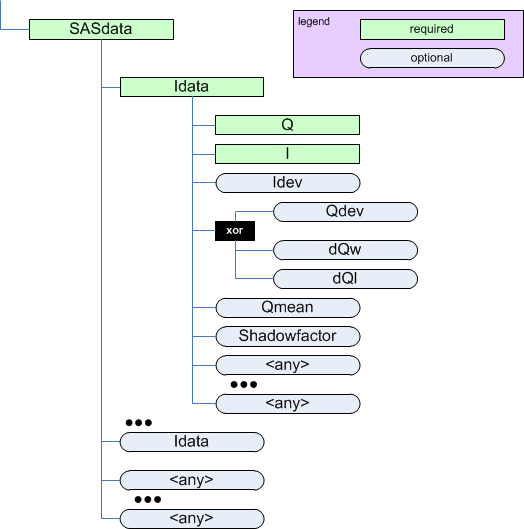SASentry

Next topic

SAStransmission_spectrum

SASdata¶

parent:
SASentryThe SASdata element

Also, see the drawing of the Q geometry.

Name Type Occurrence Description Attributes
Idata container [1..inf] Each Idata describes a single SAS data point containing $$I(Q)$$ and other terms.

Idata¶

parent:
SASdata
Name Type Occurrence Description Attributes
Q float [1..1] $$Q=(4 \pi / \lambda) \sin(\theta)$$ as defined in the Rules section. Either 1/A or 1/nm are typical units. unit={unit} 
I float [1..1] Intensity of the detected radiation. as defined in the Rules section. See the section titled The Intensity Problem for ways to describe the SAS intensity. One possibility might be 1/cm for absolute units when the intensity describes a differential cross-section per unit volume per unit solid angle. unit={unit}  
Idev float [0..1] Estimated uncertainty (usually standard deviation) of I.  It is unexpected for I and Idev to have different units. unit={unit} 
Qdev float [0..1] Estimated uncertainty (usually standard deviation) of Q.  It is unexpected for Q and Qdev to have different units. unit={unit}  
dQw float [0..1] $$Q$$ resolution along the axis of scanning (the high-resolution slit width direction). Useful for defining resolution data from slit-smearing instruments such as Bonse-Hart geometry.  It is unexpected for Q and dQw to have different units. unit={unit}  
dQl float [0..1] $$Q$$ resolution perpendicular to the axis of scanning (the low-resolution slit length direction). Useful for defining resolution data from slit-smearing instruments such as Bonse-Hart geometry.  It is unexpected for Q and dQl to have different units. unit={unit}  
Qmean float [0..1] Mean value of $$Q$$ for this data point.  Useful when describing data that has been binned from higher-resolution or from area detectors. It is unexpected for Q and Qmean to have different units. unit={unit} 
Shadowfactor float [0..1] A numerical factor applied to pixels affected by the beam stop penumbra.  
{any} container [0..inf] Any element(s) not defined in the cansas1d:1.1 standard can be placed at this point. xmlns:{foreign-prefix}={foreign-namespace}

Table Notes

  (1, 2, 3, 4, 5, 6, 7) The unit attribute is required. See Rules for acceptable values.
  Because there are several different ways to describe the SAS intensity, One should be very careful to inspect the unit attribute to determine how to handle subsequent data processing, especially in the area of units conversion.
  (1, 2, 3, 4, 5, 6) When an optional element (such as Idev, Qdev, ...) is used, it must be used in every Idata within the enclosing SASdata.
  (1, 2, 3) If either dQw or dQl are used, then Qdev is not permitted to be used.
  The Shadowfactor is used in data files from NIST/NCNR instruments. See: J.G. Barker & J.S. Pedersen (1995) J. Appl. Cryst. 28, 105-114.